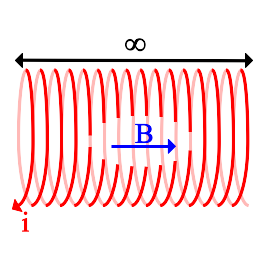# Field Inside a Straight, Infinite, Air-Core Solenoid¶

This simple formula can be derived directly from Maxwell's fourth equation, or Ampere's Law.$B = \mu_o i n$

B is the magnetic field inside the solenoid, in teslas. The direction of the field is parallel to the axis of the solenoid. There is no field outside the solenoid.

$\mathbf \mu_o$ is the permeability constant (1.26x10-6 Hm-1)

i is the current in the wire, in amperes.

n is the number of turns of wire per unit length of the solenoid, in units of $\mathrm{m^{-1}}$.

Magnet Formulas, © 2018 by Eric Dennison. Source code and License on Github